nextnano.com  GUI: nextnanomat  Tool: nextnano++  Tool: nextnano³  Tool: nextnano.NEGF  Download | Search | Copyright | News | Publications  * password protected nextnano³ softwareBIO-1D Si/SiO2 electrolyte

# nextnano3 - Tutorial

## ISFET (Ion-Selective Field Effect Transistor): Si-SiO2 electrolyte sensor

Author: Stefan Birner

If you want to obtain the input file that is used within this tutorial, please contact stefan.birner@nextnano.de.
```-> 1DSi_SiO2_electrolyte_sensor.in        - ```Loop over pH values from pH = 0 to pH = 14.``` -> 1DSi_SiO2_electrolyte_sensor_pH64.in   - ```pH = 6.4```  ```

This tutorial is based on the diploma thesis of Michael Bayer, TU Munich (2004).

Acknowledgement: The author - Stefan Birner - would like to thank Christian Uhl and Michael Bayer for helping to include the electrolyte features into nextnano³.

## Si-SiO2 electrolyte sensor

Here, we predict the sensitivity of electrolyte gate Si-SiO2 sensors to pH values of the electrolyte solution that covers the semiconductor structure.
The charge density due to chemical reactions at the oxidic semiconductor-electrolyte interface is described within the site-binding model (`\$interface-states`).
We calculate the spatial charge and potential distribution both in the semiconductor and the electrolyte (Poisson-Boltzmann equation) self-consistently.

The Si-SiO2 sensor that is exposed to an electrolyte solution has the following schematic layout: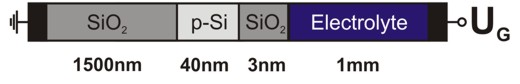Fig. 1: Si-SiO2 sensor with electrolyte gate.

We simulate the structure along the z direction so that the structure is effectively one-dimensional, i.e. laterally homogeneous.
The heterostructure is assumed to be grown along the  direction and is not strained.

The file``` densities/interface_densitiesD.txt ```gives us information about the relevant interface charge densities:

• Interface 4 (1544 nm): Interface charge sigmaadsorbed that results from the site-binding model that describes chemical reactions at the oxidic semiconductor-electrolyte interface. More details: ``` \$interface-states```
``` \$interface-states   state-number          = 1           ! between SiO2 / Electrolyte at 1544 nm   state-type            = electrolyte ! sigma_adsorbed   interface-density     = 5.0d14      ! [cm^-2] - ```total density of surface sites, i.e. surface hydroxyl groups```   adsorption-constant   = 0.079433d0  ! K1 = adsorption   constant = 1 * 10-1.1   dissociation-constant = 2.511886d-5 ! K2 = dissociation constant = 1 * 10-4.6  \$electrolyte   ...   pH-value              = 6.4d0       ! pH = -lg(concentration) = 6.4 -> ```concentration in``` [M]=[mol/l] ``````   ```(The point of zero charge for SiO2 is at pH = 2.2.)

The Si region (1501 nm - 1541 nm) is homogeneously p-type doped with boron having a concentration of 1 * 1016 cm-3.

The electrolyte region (1544 nm - 9999 nm) contains the following ions:
```  !---------------------------------------------------------------------------!  ! The electrolyte (phosphate buffer, NaCl) contains five types of ions:  !   1)   7 mM singly charged anions  (1-)     <- 10 mM phosphate buffer (PBS) H2PO4-  !   2)   3 mM doubly charged anions  (2-)     <- 10 mM phosphate buffer (PBS) HPO42-  !   3)  13 mM singly charged cations (1+)     <- 10 mM phosphate buffer (PBS) Na+  !   4)  10 mM singly charged cations (Na+)    <- 10 mM NaCl  !   5)  10 mM singly charged anions  (Cl-)    <- 10 mM NaCl  !---------------------------------------------------------------------------!  \$electrolyte-ion-content   ion-number        = 1              ! ```7 mM singly charged anions```   ion-valency       = -1d0           ! ```charge of the ion:``` 1-   ion-concentration =  7d-3          ! ```Input in units of:``` [M] = [mol/l] = 1d-3 [mol/cm³]   ion-region        = 1544d0  9999d0 ! ```refers to region where the electrolyte has to be applied to```   ion-number        = 2              ! ```3 mM doubly charged anions```   ion-valency       = -2d0           ! ``` charge of the ion:``` 2-   ion-concentration =  3d-3          ! ```Input in units of:``` [M] = [mol/l] = 1d-3 [mol/cm³]   ion-region        = 1544d0  9999d0 ! ```refers to region where the electrolyte has to be applied to```   ion-number        = 3              ! ```13 mM singly charged cations```   ion-valency       =  1d0           ! ``` charge of the ion:``` 1+   ion-concentration = 13d-3          ! ```Input in units of:``` [M] = [mol/l] = 1d-3 [mol/cm³]   ion-region        = 1544d0  9999d0 ! ```refers to region where the electrolyte has to be applied to```   ion-number        = 4              ! ```10 mM singly charged cations```   ion-valency       =  1d0           ! ``` charge of the ion:``` Na+   ion-concentration = 10d-3          ! ```Input in units of:``` [M] = [mol/l] = 1d-3 [mol/cm³]   ion-region        = 1544d0  9999d0 ! ```refers to region where the electrolyte has to be applied to```   ion-number        = 5              ! ```10 mM singly charged anions```   ion-valency       = -1d0           ! ```charge of the ion:``` Cl-   ion-concentration = 10d-3          ! ```Input in units of:``` [M] = [mol/l] = 1d-3 [mol/cm³]   ion-region        = 1544d0  9999d0 ! ```refers to region where the electrolyte has to be applied to

In addition to these five types of ions, the pH value (as specified in` \$interface-states`) automatically determines inside the code four further types of ions, namely the concentration of H3O+, OH`-` and the corresponding anions`-` (conjugate base: [anion`-`] = 10-pH - 10-pOH = 10-6.4 - 10-7.6 = 3.73 x 10-7) and cations+ (conjugate acid; zero in this tutorial for pH = 6.4 because pH = 6.4 < 7). For details, confer` \$electrolyte-ion-content`.

We have to solve the nonlinear Poisson equation over the whole device, i.e. including the Poisson-Boltzmann equation that governs the charge density in the electrolyte region.
As for the boundary conditions we assume at the right boundary (Electrolyte/Metal) a Dirichlet boundary condition where the electrostatic potential phi is equal to UG where UG is the gate voltage determined by an electrode in the electrolyte solution and which is constant throughout the entire electrolyte region. In this example the applied gate voltage is UG = 0 V, i.e. the electrostatic potential in the Poisson-Boltzmann equation is fixed to 0 V. Note that the reference potential UG enters the Poisson-Boltzmann equation and also the equation for the site-binding model at the oxide/electrolyte interface. So the Dirichlet boundary condition is phi = 0 V. This corresponds to the fact that at the right part of the electrolyte,  i.e. at 'infinity' (at 9999 nm) the ion concentration is the 'equilibrium' (default) concentration as defined in``` \$electrolyte-ion-content```.
At the left boundary (Metal/SiO2) we use a Neumann boundary condition with a zero potential gradient that corresponds to an electric field E = 0 V/m.

```  \$poisson-boundary-conditions    poisson-cluster-number  = 1    region-cluster-number   = 1    boundary-condition-type = Neumann    electric-field          = 0d0      ! 0 [V/m]    poisson-cluster-number  = 2    region-cluster-number   = 6    boundary-condition-type = Dirichlet    potential               = 0d0      ! phi = 0 [V] <=> UG = 0 [V]```

## Oxide/electrolyte interface potential as a function of pH value

The Si-SiO2 heterostructure acts as a sensor via the semiconductor-electrolyte interface potential that reflects sigmaadsorbed, the pH value and the spatial dependence of the electrostatic potential in the solution as described by the Poisson-Boltzmann theory.

Choosing` flow-scheme = 30`, several calculations are performed while sweeping over the pH value from 0 to 14.
```    pH-value              = ```(value is overwritten internally in the program)

The file` InterfacePotentialDensity_vs_pH1D.dat `gives us the information about the electrostatic potential at the oxide/electrolyte interface for different pH values.

The surface potential is defined as the difference of the electrostatic potential at the oxide/electrolyte interface and the reference potential UG (Here: UG = 0 V).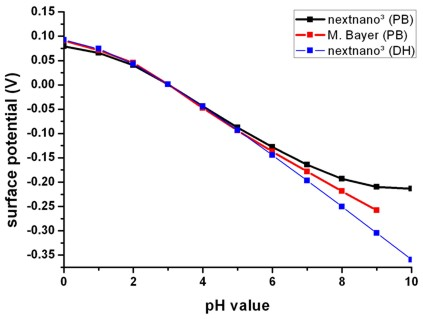Fig. 2: Calculated oxide/electrolyte interface potential as a function of the pH value by using the nonlinear Poisson-Boltzmann (PB) ion distribution.
For comparison the results obtained using the linear Debye-Hückel (DH) ion distribution are also shown.
As expected, in the case of zero surface potential, the Poisson-Boltzmann and the Debye-Hückel results are identical.
(Electrolyte: 10 mM phosphate buffer, 10 mM NaCl)

The slope (d phi / d pH) is a characteristic property of an oxide/electrolyte interface. Note that in Fig. 2 only the slope is relevant but not the absolute values of the potential. A linear fit between pH = 0 and pH = 9 yields 37.7 mV/pH in agreement with experiments (P. Bergveld, A. Sibbald, Analytical and biomedical applications of ion-selective-field-effect-transistors, ed. G. Svehla, Analytical Chemistry, Vol. XXIII, Elsevier, 1988).

Further below (Fig. 3) we see that the oxide/electrolyte interface charge density saturates at high pH values (pH = [10,...,14]). This also means that this charge density is then (almost) unaffected by the oxide/electrolyte interface potential. Thus this quantity is then determined purely by the Poisson-Boltzmann ion distribution in the electrolyte, i.e. by the concentration of OH`-` (and corresponding cations+) that are the most present ion species at high pH values.

## Oxide/electrolyte interface charge density sigmaadsorbed as a function of pH value

From the file` InterfacePotentialDensity_vs_pH1D.dat `we also obtain information about the oxide/electrolyte interface sheet charge density sigmaadsorbed (as a function of pH value) that is determined by the amphoteric reactions at the oxide surface.

We plot in Fig. 3 the oxide/electrolyte interface charge density sigmaadsorbed: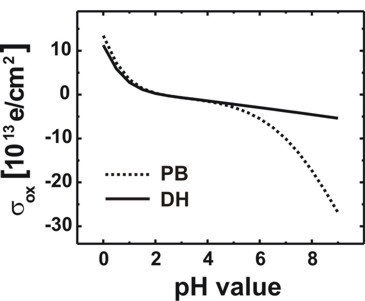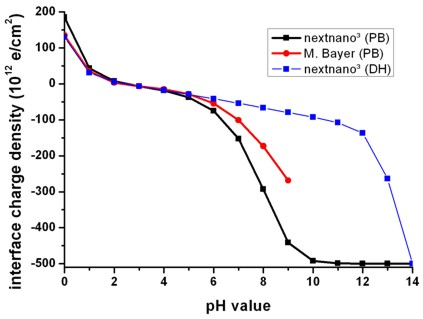Fig. 3: Calculated variation of the oxide/electrolyte interface charge density sigmaadsorbed of the amphoteric oxide surface with the pH value of the electrolyte solution by using the nonlinear Poisson-Boltzmann (PB) ion distribution.
Note that there is a range of pH values where the net surface charge density is close to zero.
The calculated point of zero charge for the SiO2 surface is reached for pH = 2.2.
For comparison the results obtained using the linear Debye-Hückel (DH) ion distribution are also shown.

At high pH values the interface charge density saturates at the nominal density of surface sites because at the oxide/electrolyte interface all surface sites adsorbed the hydrogen ions from the electrolyte solution.```  interface-density = 5.0d14 ! [cm^-2]    => sigmaadsorbed = 500 * 1012 [e/cm2] ```Thus for pH values ranging from 10 to 14, the oxide/electrolyte interface charge density is (almost) independent of both the pH value and the electrostatic potential.

## Electrostatic potential for electrolyte gate voltage UG = 0

Here we plot the electrostatic potential for a pH value of 6.4.
UG = 0 V, i.e. we apply a zero gate voltage to the electrolyte. Note that UG is both the Dirichlet boundary condition for the electrostatic potential at the right contact as well as the reference potential that enters into the Poisson-Boltzmann equation (i.e. into the exponential term of the ion charge density). UG is constant throughout the entire electrolyte region.

UG =   0 V:
```   poisson-cluster-number  = 2    region-cluster-number   = 6    boundary-condition-type = Dirichlet    potential               = 0d0      ! phi =  0 [V] <=> UG =  0 [V]```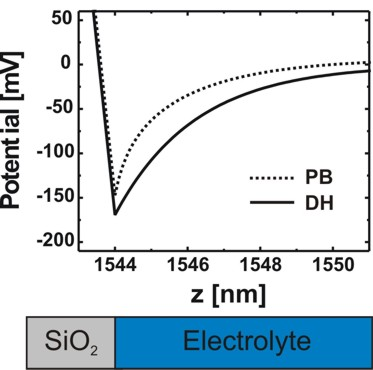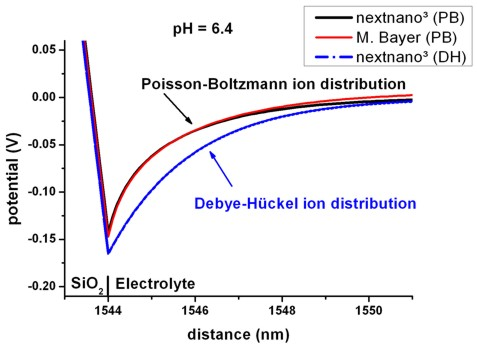Fig. 4: Spatial electrostatic potential distribution for pH = 6.4 in the electrolyte by using the nonlinear Poisson-Boltzmann (PB) ion distribution.
For comparison the results obtained using the linear Debye-Hückel (DH) ion distribution are also shown.
(Electrolyte: 10 mM phosphate buffer, 10 mM NaCl, UG = 0 V)

• Please help us to improve our tutorial. Send comments to``` support [at] nextnano.com```.